### Abstract

Amplitude-shift keying (ASK) and on-off keying (OOK) receivers are used for intermittent low-data-rate applications like RKE, home security, garage-door openers, and remote controls. The data that comes to an ASK or OOK receiver from a remote transmitter is reconstructed in the data slicer. The data slicer is, therefore, an integral part of ASK and FSK receivers that operate in the 260MHz to 470MHz short-range UHF band under the rules of FCC Part 15.231. This application note explains the operation of the data slicers found in Maxim's UHF receivers, including the MAX1470, MAX1473, and MAX1471, and transceivers like the MAX7030 and MAX7032.

### Introduction

In its simplest form, the data slicer is an analog comparator that compares the demodulated ASK signal with a threshold. If the demodulated signal voltage exceeds the threshold, the comparator output goes high, usually to the supply voltage. If the demodulated signal goes below the threshold, the comparator output goes low, usually zero volts or ground.

This application note reviews two aspects of data slicing: forming the comparator threshold, and preventing the comparator output from 'chattering' when no signal is present. The latter operation, often called 'squelching', can be done by introducing simple voltage offsets onto either pin of the data comparator. This offset can come directly from the power supply or from using hysteresis, which is the process of feeding back part of the output voltage from the data slicer comparator.

We will show three different ways to form the threshold, and three different ways to introduce squelch, all of which can be done by adding a few external resistors and/or capacitors.

The Maxim ASK receivers use a demodulator that is a carefully designed limiting IF amplifier. This amplifier produces a voltage that is proportional to the logarithm of the input IF signal power. When no signal is present, the voltage formed by the amplifier consists of a quiescent DC value with a small time-varying noise voltage riding on it. Figure 1 shows the waveform for the demodulator output in response to an ASK-modulated signal. The waveform will go back and forth between the quiescent voltage, V0, when the signal is keyed off, and the signal voltage, Vs, when the signal is keyed on. In the MAX1473, V0 is typically about 1.2V and Vs ranges from about 40mV at sensitivity to about 1V at very high signal levels.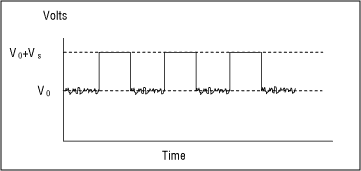### Data Slicer Block Diagrams

Figure 2 shows a block diagram of the MAX1473 ASK receiver. This application note focuses on the three operational amplifiers and the seven pins at the bottom right of the diagram that comprise the data slicer. The same functional blocks are redrawn in Figure 3 to illustrate the function of each circuit more clearly. The reference designators for the resistors and capacitors in these figures are the same as those in the MAX1473 Evaluation Kit schematic. The operational amplifier, U1, and its components form the Sallen-Key data filter, which smoothes the output of the detected amplitude from the ASK demodulator. The operational amplifier, U2, and its components form the data slicer comparator, while the peak-detecting operational amplifier, U3, and its components form the peak-detector output. We can now focus on individual parts of this circuit to understand the various options within the data-slicing operation.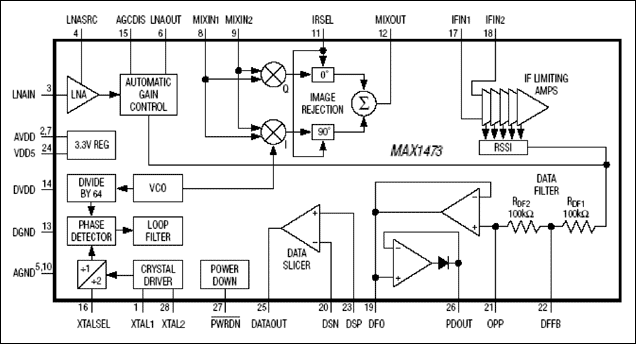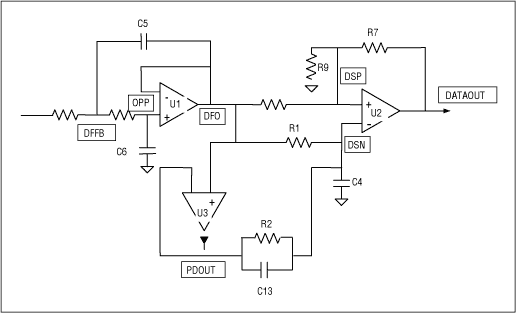Figure 3. Block diagram of the data-slicer blocks in the MAX1473, including external components.

### The Fundamental Data-Slicing Circuit

Figure 4 shows the simplest data-slicing circuit. The output of the data filter, DFO, goes to the positive pin of the data slicer comparator, DSP, and also forms the slicing threshold voltage at DSN by passing through a simple RC lowpass filter. When the detected and filtered ASK signal, DFO, passes through the lowpass filter formed by R1 and C4 and settles, the DC value of the DSN pin is halfway between the maximum and minimum voltage of that signal. Figure 5 illustrates the waveforms at DSP and DSN, using the voltages V0 and Vs from Figure 1. Notice that the steady-state voltage at DSN is V0 + Vs/2. This circuit functions well when the received data stream has enough extra bits at the beginning of a packet or frame (in the form of a preamble or synch pattern) that it can afford to lose while the R1-C4 circuit charges up to the correct slicing threshold. When the first bits of a sequence need to be detected, the circuit that forms the threshold voltageat DSN needs to reach that voltage quickly. This is where the peak detector can help.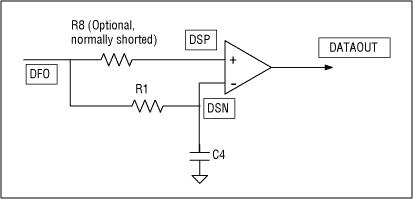Figure 4. Fundamental data-slicing circuit.Figure 5. DSN and DSP signal for fundamental data-slicing threshold formation.

### Data Slicer with Rapid Threshold Formation

Adding a voltage from the peak detector in the ASK receivers can accelerate the formation of the data-slicing threshold DSN. The circuit in Figure 6 illustrates how the contributions to DSN from the data filter and the peak detector combine to produce a rapidly responding threshold voltage. By considering DFO and PDOUT as two independent voltage sources, we can use the Superposition technique (find the response from each source alone, then add the responses) to determine the voltage at DSN. The contribution from the peak detector is an instantaneous voltage jump through the capacitive divider formed by C13 and C4. This voltage jump decays to a steady-state value determined by the resistive divider formed by R1 and R2. The contribution from the R1-C4 lowpass filter is the same slowly rising threshold from the fundamental data-slicer circuit. By choosing the two R and two C values carefully, the two contributions can complement one another and form a threshold voltage at DSN that ideally jumps immediately to the correct threshold and stays there.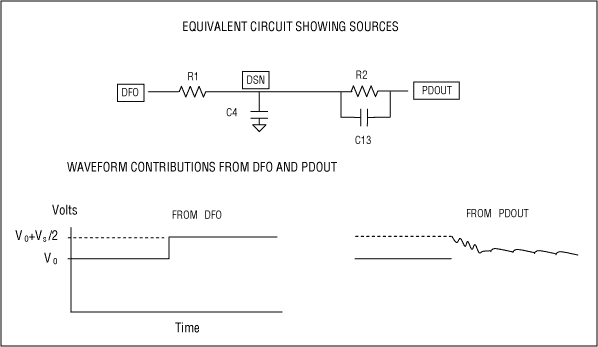Figure 6. Circuit and waveforms for rapid threshold formation.

Figure 7 illustrates two DSN waveforms for two different sets of resistors and capacitors. The combination of components that produces a threshold voltage at DSN closest to an instantaneous jump obeys the guideline below:Figure 7. Combined DSN voltage vs. time using a peak detector.

We can illustrate the choice of the R's and C's with a specific example. For an ASK data rate of 4kbps NRZ, the R1-C4 lowpass filter should have a time constant of about 5-bit intervals, which is 5 x 0.25ms, or 1.25ms. A good choice of R1 and C4 is:

R1 = 25 kΩ and C4 = 0.047 µF

We choose C13 equal to C4, and make R2 much larger than R1 (a factor of 10 is good):

R2 = 250 kΩ and C13 = 0.047 µF

This choice will cause the threshold voltage DSN to jump from V0 to V0 + Vs/2, then settle to V0 + 0.55Vs.

Notice that this approach to establishing a rapid slicing threshold creates a small error in the threshold. Moreover, the time constant associated with the threshold voltage's change from its initial to final value, which is a very small change, is given by the product below:

Time Constant = (R1 || R2 x (C4 + C13))

This is approximately twice the time constant of the R1-C4 smoothing circuit. We could correct for this change by reducing each capacitor value, but that is not necessary. Because the threshold changes very little after its initial jump, the time constant is not as critical as it is in a circuit that lacks the peak-detector contribution.

### Data Slicer with Dual Peak Detectors

There is a minor drawback to using the single peak detector in combination with the R-C smoothing circuit to form the slicing threshold: the final threshold value differs slightly from its ideal value which is halfway between the maximum and minimum voltage that comes from the data filter.

Using a maximum and minimum peak detector is one way to improve the rapid establishment of the slicing threshold. The MAX1471 ASK/FSK receiver, the MAX7042 FSK receiver, and the MAX7030/MAX7031/MAX7032 transceivers have maximum and minimum peak detectors so that the single R-C smoothing circuit is not needed. Figure 8 shows these peak detectors, each with an external resistor and capacitor. Each capacitor holds the peak voltage, and each resistor provides a discharge path for the associated capacitor. This design allows the peak detectors to dynamically follow any peak changes in the data-filter output voltages. The maximum and minimum peak detectors can be used together to form a data-slicer threshold voltage at a value midway between the maximum and minimum voltage levels of the data stream. The RC time constant of these R-C pairs should be set to about five times the bit interval, as it is in the simple threshold smoothing circuit described earlier in this application note.Figure 8. Data-slicer circuit with maximum and minimum peak detectors.

If something causes a significant change in the magnitude of the baseband signal, such as an AGC gain switch or a power-up transient, the peak detectors may 'catch' a false level. If a false peak is detected, the slicing level is incorrect. Because the RC time constants are several bits long, the peak detectors may not recover rapidly. The Maxim receivers with dual peak detectors, however, all have at least one provision for resetting the peak detector outputs: the receivers momentarily allow the peak detectors to track the signal. In the MAX7042 FSK receiver, the peak detectors are reset by momentarily pulling the ENABLE pin low, then returning it to a logical high setting. The MAX7030 and MAX7031 transceivers reset the peak detectors the same way, but also reset the peak detectors whenever the AGC function changes state or the T/R switch enters the receive state. The MAX1471 ASK/FSK receiver and the MAX7032 ASK/FSK transceiver reset the peak detectors through their serial ports, and also automatically reset the peak detectors whenever the receiver emerges from the sleep mode.

### Adding Basic Squelch to the Data Slicer

In the absence of an ASK signal, the ASK detector output consists of a DC voltage with a time-varying noise voltage whose peak-to-peak value is about 20mV. This noise voltage appears as the data-slicer comparator swings back and forth across the DSN threshold voltage, causing the comparator's output to 'chatter', that is, to jump rapidly and randomly back and forth between the supply voltage and ground. This behavior often wakes up microprocessors unnecessarily and can sometimes add noise to the power-supply lines. One way to stop this chatter is to use a simple squelch circuit, which adds a small DC offset to either the positive (DSP) or negative (DSN) pin of the data slicer.

Figure 9 shows simple squelch circuits that use the power supply as the source of the DC offset. Usually, all you need is a large resistor that is 50 to 100 times the value of the resistor between the data-filter output DFO and either input pin on the comparator. In the first circuit of Figure 9, the small offset is added to DSP. If the offset is about 30mV, then two things will happen. Firstly, the noise riding on the DC voltage at DSP in the absence of a signal will never take the DSP voltage below the level of the threshold at DSN; and, secondly, the DATAOUT pin will be held high, i.e., VDD. In the second circuit of Figure 9, the offset is added to DSN. Now the noise that rides on the DC voltage at DSP will never take the DSP voltage above the increased threshold at DSN and the DATAOUT pin will be held low, i.e., GND. The squelch circuit reduces sensitivity slightly (about 1dB to 2dB when the resistive divider is chosen carefully), and causes a slightly wider positive data pulse and slightly narrower negative data pulse at DATAOUT when a demodulated signal is present.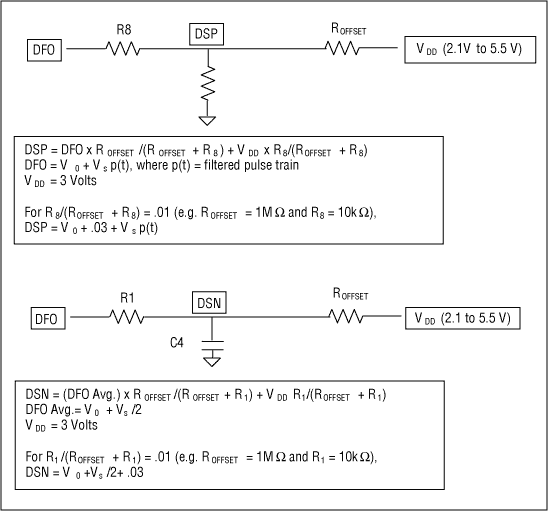Figure 9. Two simple squelch circuits using a supply voltage.

### Squelch from Dual Peak Detectors

Another simple squelch circuit can be formed by using the dual peak detectors in Figure 8 above. Making the two resistors slightly unequal will shift the threshold above or below the midpoint of the two peak voltages, depending on which resistor is larger. If the threshold is set slightly above the midpoint by 30mV to 50mV, the DATAOUT pin will stay low when no signal is present. Similarly, if the threshold is set slightly below the midpoint, the DATAOUT pin will stay high when no signal is present.

### Adding Resistive Hysteresis to the Data Slicer

You can also use a large resistor to tie the DATAOUT pin from the data slicer to the DSP pin . Figure 10 shows the equivalent circuit for resistive hysteresis. This approach has almost the same effect as connecting VDD to the DSP pin through a resistor. The only difference here is that, when a demodulated signal is present, the small offset at DSP is there only during the positive swing of the demodulated data. Hence, the increase in the width of the positive data pulse at DATAOUT is slightly less, because the leading edge of the positive data pulse is not advanced by the presence of an offset.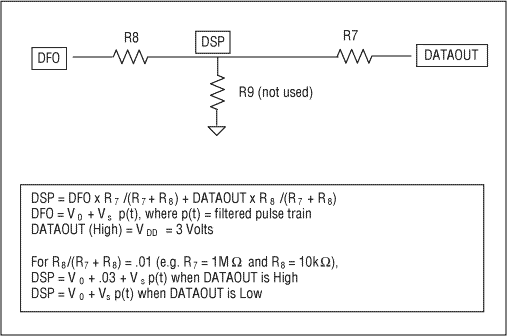Figure 10. Resistive hysteresis circuit for squelch function.

### Adding Capacitive Hysteresis to the Data Slicer

Capacitive hysteresis offers a compromise between excessive chattering of the DATAOUT signal and the reduced sensitivity that comes from a squelch or resistive hysteresis. The circuit for capacitive hysteresis is shown in Figure 11.

Just as in resistive hysteresis, a small fraction of the DATAOUT signal is fed back to the DSP pin, this time through the capacitive divider C7-C9. Typical capacitor values are 10pf for C7 and 1000pf for C9. The offset added to DSP differs because it is a transient offset that decays with a time constant given by:

R8 x (C9 + C7)

Depending on the length of the time constant, the offset keeps the noise on DSP from going below the slicing threshold until the offset decays. This effectively increases the time that the DATAOUT pin stays high, thereby reducing the frequency of the DATAOUT chatter. While capacitive hysteresis does not eliminate chatter altogether, it reduces the number of transitions.

Notice that the presence of C9 creates another lowpass filter with R8 in the demodulated ASK signal path. The pole associated with this filter time constant should be larger than the bandwidth of the Sallen-Key data filter, so that it does not make the filtered signal too sluggish.Figure 11. Capacitive hysteresis circuit and waveforms.